## Probability Function

The probability density function(also called the Probability Density Function) of a continuous distribution is defined as the derivative of the (cumulative) Distribution Function,(1)

so(2)

A probability density function satisfies(3)

and is constrained by the normalization condition,(4)

Special cases are(5)(6)(7)

If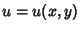and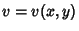, then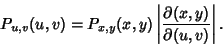(8)

Given the Moments of a distribution (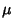,, and the Gamma Statistics), the asymptotic probability function is given by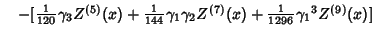(9)
where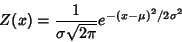(10)

is the Normal Distribution, and(11)

for(withCumulants andthe Standard Deviation; Abramowitz and Stegun 1972, p. 935).

See also Continuous Distribution, Cornish-Fisher Asymptotic Expansion, Discrete Distribution, Distribution Function, Joint Distribution Function

References

Abramowitz, M. and Stegun, C. A. (Eds.). Probability Functions.'' Ch. 26 in Handbook of Mathematical Functions with Formulas, Graphs, and Mathematical Tables, 9th printing. New York: Dover, pp. 925-964, 1972.